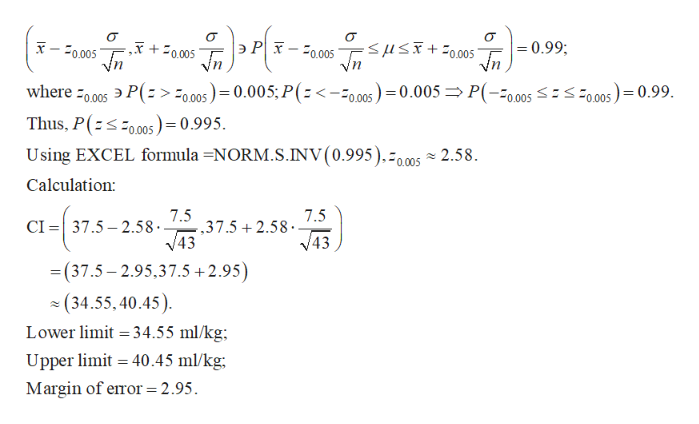# Total plasma volume is important in determining the required plasma component in blood replacement therapy for a person undergoing surgery. Plasma volume is influenced by the overall health and physical activity of an individual. Suppose that a random sample of 43 male firefighters are tested and that they have a plasma volume sample mean of x = 37.5 ml/kg (milliliters plasma per kilogram body weight). Assume that σ = 7.50 ml/kg for the distribution of blood plasma.(a) Find a 99% confidence interval for the population mean blood plasma volume in male firefighters. What is the margin of error? (Round your answers to two decimal places.)lower limit    1upper limit    2margin of error    3(b) What conditions are necessary for your calculations? (Select all that apply.)n is largethe distribution of weights is normalσ is knownthe distribution of weights is uniformσ is unknown(c) Interpret your results in the context of this problem.1% of the intervals created using this method will contain the true average blood plasma volume in male firefighters.The probability that this interval contains the true average blood plasma volume in male firefighters is 0.99.     The probability that this interval contains the true average blood plasma volume in male firefighters is 0.01.99% of the intervals created using this method will contain the true average blood plasma volume in male firefighters.(d) Find the sample size necessary for a 99% confidence level with maximal margin of error E = 2.50 for the mean plasma volume in male firefighters. (Round up to the nearest whole number.) 6 male firefighters

Question
848 views

Total plasma volume is important in determining the required plasma component in blood replacement therapy for a person undergoing surgery. Plasma volume is influenced by the overall health and physical activity of an individual. Suppose that a random sample of 43 male firefighters are tested and that they have a plasma volume sample mean of x = 37.5 ml/kg (milliliters plasma per kilogram body weight). Assume that σ = 7.50 ml/kg for the distribution of blood plasma.

(a) Find a 99% confidence interval for the population mean blood plasma volume in male firefighters. What is the margin of error? (Round your answers to two decimal places.)
 lower limit 1 upper limit 2 margin of error 3

(b) What conditions are necessary for your calculations? (Select all that apply.)
n is large
the distribution of weights is normal
σ is known
the distribution of weights is uniform
σ is unknown

(c) Interpret your results in the context of this problem.
1% of the intervals created using this method will contain the true average blood plasma volume in male firefighters.
The probability that this interval contains the true average blood plasma volume in male firefighters is 0.99.
The probability that this interval contains the true average blood plasma volume in male firefighters is 0.01.
99% of the intervals created using this method will contain the true average blood plasma volume in male firefighters.
(d) Find the sample size necessary for a 99% confidence level with maximal margin of error E = 2.50 for the mean plasma volume in male firefighters. (Round up to the nearest whole number.)
6 male firefighters
check_circle

Step 1

Note:

Hi! Thank you for posting the question. Since your question has more than 3 parts, we have solved the first 3 parts for you. If you need help with any other parts, please re-post the question and mention the part you need help with.

Step 2

(a) Calculation of confidence interval:

The 99% confidence interval (CI) of the mean for a large sample (sample size, n greater than 30) for known standard deviation, σ is obtained as follows:help_outlineImage TranscriptioncloseSO00-- Vn =0.99; . Vn where 0,005P(> 00= 0.005; P(:<-0.005 0.005 > P(-20,005 s 0,00s)= 0.99 Thus, P(s00)= 0.995 Using EXCEL formula =NORM.S.INV(0.995). -005 2.58. Calculation 0.005 7.5 .,37.52.58 V43 7.5 CI 37.5 2.58 V43 (37.5-2.95,37.5+2.95) (34.55,40.45 Lower limit 34.55 ml/kg Upper limit 4045 ml/kg Margin of error 2.95 fullscreen
Step 3

(b) Conditions:

In order to ensure that the confidence interval obtained using the above formula is valid, the following conditions must be satisfied:

• The sample size must be large (n ≥ 30) or the distribution of the variable of interest must be at least approximately normal.
• The true population standard deviation must be known.

Thus, the conditions...

### Want to see the full answer?

See Solution

#### Want to see this answer and more?

Solutions are written by subject experts who are available 24/7. Questions are typically answered within 1 hour.*

See Solution
*Response times may vary by subject and question.
Tagged in

### Statistics